### Python稀疏矩阵

• 1. 导入模块
• 2. SciPy中的稀疏矩阵
• 2.1 坐标列表格式 COO
• 2.2 格式转换
• 2.3 压缩列格式和压缩行格式 CSR/CSC
• 3. 创建稀疏矩阵
• 3.1 稀疏矩阵的可视化
• 3.2 稀疏矩阵线性代数
• 3.3 线性方程组
• 3.4 LU分解
• 3.5 特征值问题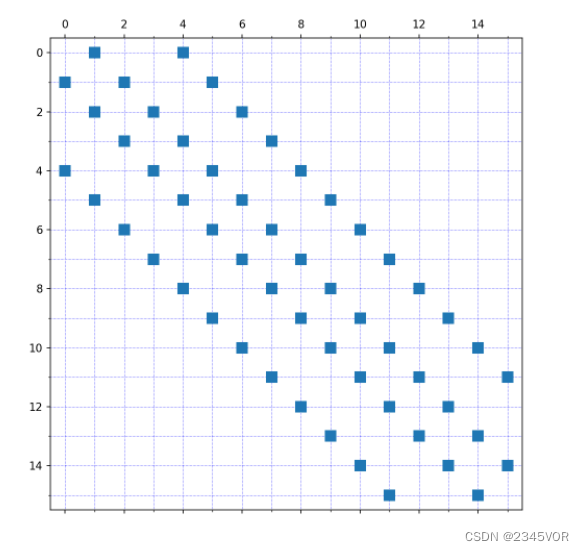## 1. 导入模块

SciPy中提供了稀疏矩阵模块scipy.sparse，为稀疏矩阵的表示及其线性代数运算提供了丰富易用的接口。

import matplotlib.pyplot as plt
import matplotlib as mpl

import numpy as np

import scipy.sparse as sp
import scipy.sparse.linalg

import scipy.linalg as la

• 1
• 2
• 3
• 4
• 5
• 6
• 7
• 8
• 9

## 2. SciPy中的稀疏矩阵

### 2.1 坐标列表格式 COO

A

=

[

0

1

0

0

0

0

0

2

0

0

3

0

4

0

0

0

]

A =

A=0 1 0 00 0 0 20 0 3 04 0 0 0

values = [1, 2, 3, 4]
rows = [0, 1, 2, 3]
cols = [1, 3, 2, 0]
A = sp.coo_matrix((values, (rows, cols)), shape=[4, 4])
A

• 1
• 2
• 3
• 4
• 5SciPy的sparse模块中稀疏矩阵的属性大部分派生自NumPy的ndarray对象，同时也包括nnz（非零元素数目）和data（非零值）等属性。

A.shape, A.size, A.dtype, A.ndim

• 1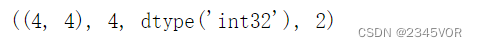A.nnz, A.data

• 1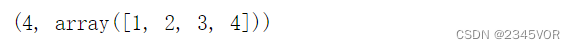A.row, A.col

• 1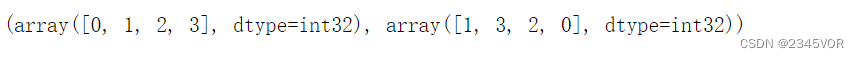### 2.2 格式转换

A.todense()  # 转换为稠密矩阵

• 1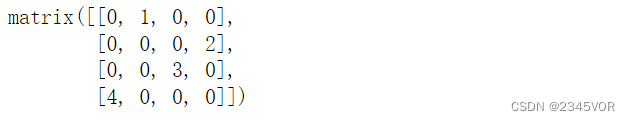A.toarray()  # 转换为ndarray数组

• 1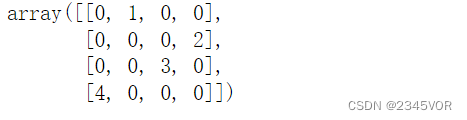A.tocsr()  # 转换为CSR格式

• 1try:
A.tobsr()  # 块稀疏矩阵不支持索引
except NotImplementedError:
print("NotImplementedError")

• 1
• 2
• 3
• 4### 2.3 压缩列格式和压缩行格式 CSR/CSC

A = np.array([[1, 2, 0, 0], [0, 3, 4, 0], [0, 0, 5, 6], [7, 0, 8, 9]])
A

• 1
• 2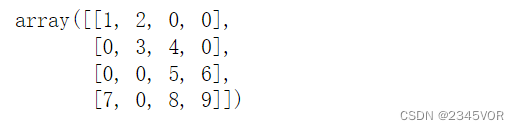A = sp.csr_matrix(A)
A.data  # 所有非零元素

• 1
• 2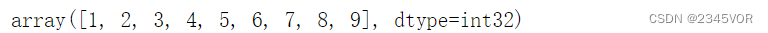A.indices # 非零元素列序号

• 1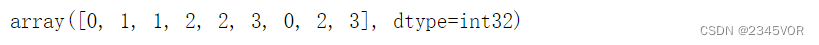A.indptr  # 每一行第一个非零元素在data中的起始序号

• 1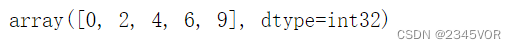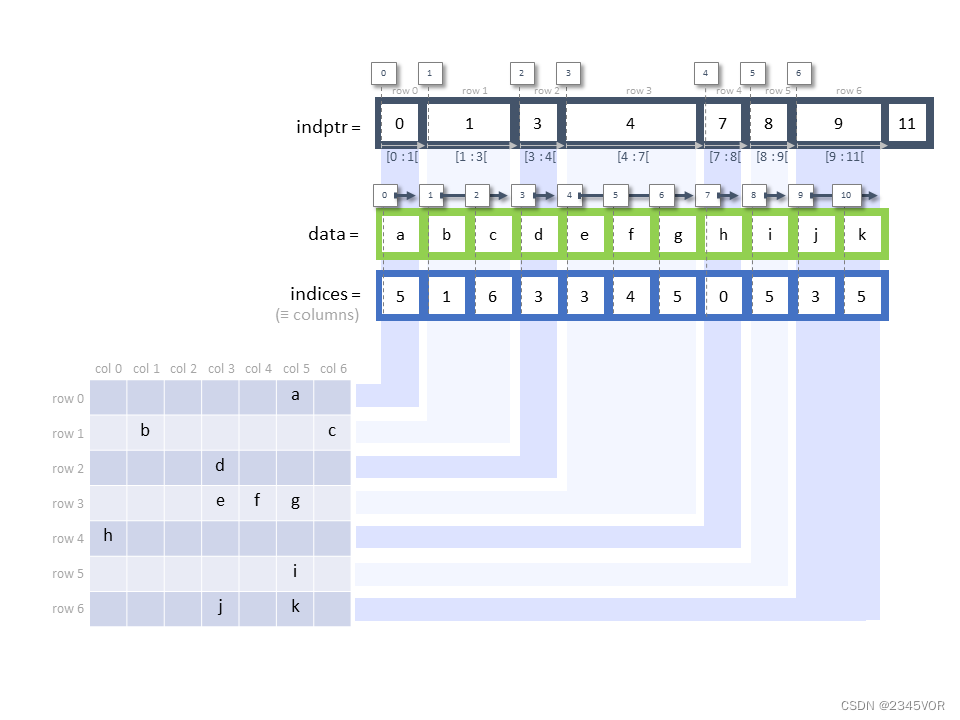## 3. 创建稀疏矩阵

sp.eye用于生成对焦矩阵，sp.kron用于计算两个稀疏矩阵的Kronecker张量积，bmatvstackhstack用于从稀疏块矩阵、垂直和水平堆叠矩阵来生成稀疏矩阵。

N = 10
A = -2 * sp.eye(N) + sp.eye(N, k=1) + sp.eye(N, k=-1)
print(type(A))
A.todense()

• 1
• 2
• 3
• 4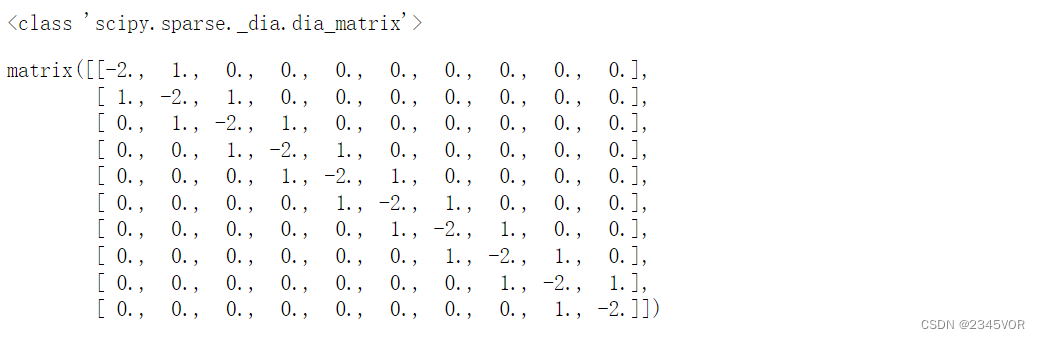A = sp.diags([1,-2,1], [1,0,-1], shape=[N, N], format='csc')
A

• 1
• 2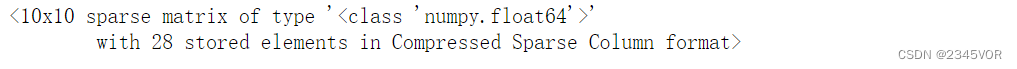d

2

f

d

x

2

=

lim

h

0

f

(

x

h

)

2

f

(

x

)

+

f

(

x

+

h

)

h

2

\frac{d^{2} f}{d x^{2}}=\lim _{h \rightarrow 0} \frac{f(x-h)-2 f(x)+f(x+h)}{h^{2}}

dx2d2f=h0limh2f(xh)2f(x)+f(x+h)

d

2

f

d

x

2

=

[

2

1

0

0

0

1

2

1

0

0

0

1

2

0

0

0

0

0

2

1

0

0

0

1

2

]

[

f

(

a

)

f

(

a

+

h

)

f

(

a

+

2

h

)

f

(

a

+

(

n

2

)

h

)

f

(

b

)

]

/

h

2

=

Lap

f

\frac{d^{2} f}{d x^{2}}=

$\left[\begin{array}{c}\begin{array}{cccccc}-2& 1& 0& \dots & 0& 0\\ 1& -2& 1& \dots & 0& 0\\ 0& 1& -2& \dots & 0& 0\\ \dots & \dots & \dots & \dots & \dots & \dots \\ 0& 0& 0& \dots & -2& 1\\ 0& 0& 0& \dots & 1& -2\end{array}\end{array}\right]$

$\left[\begin{array}{c}\begin{array}{c}f\left(a\right)\\ f\left(a+h\right)\\ f\left(a+2h\right)\\ \dots \\ f\left(a+\left(n-2\right)h\right)\\ f\left(b\right)\end{array}\end{array}\right]$

/ h^{2}=\operatorname{Lap}|f\rangle

dx2d2f=2100012100012000002100012f(a)f(a+h)f(a+2h)f(a+(n2)h)f(b)/h2=Lapf

### 3.1 稀疏矩阵的可视化

matplotlib提供的spy函数可以对稀疏矩阵进行可视化。

fig, ax = plt.subplots()
ax.spy(A)

• 1
• 2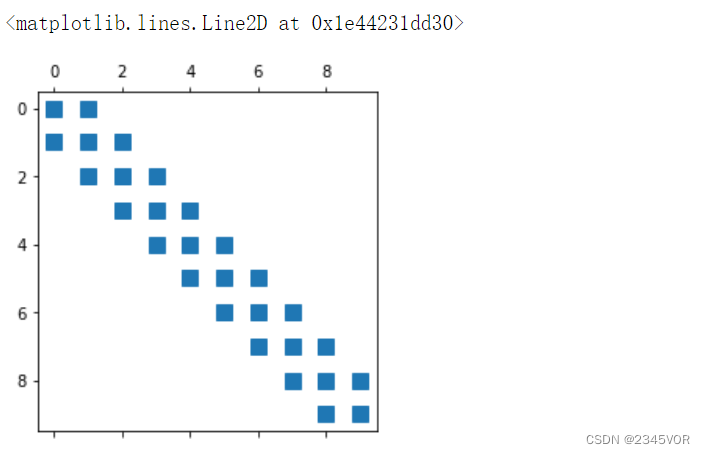B = sp.diags([1, 1], [-1, 1], shape=[3,3])
B.todense()

• 1
• 2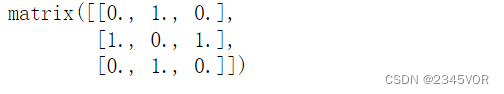C = sp.kron(A, B, format='csr')

fig, (ax_A, ax_B, ax_C) = plt.subplots(1, 3, figsize=(12, 4))
ax_A.spy(A)
ax_B.spy(B)
ax_C.spy(C)

• 1
• 2
• 3
• 4
• 5
• 6### 3.3 线性方程组

A

x

=

b

Ax=b

Ax=b形式的线性方程组，以前面提到的三对角矩阵为例：

N = 10
A = sp.diags([1, -2, 1], [1, 0, -1], shape=[N, N], format='csc')
b = -np.ones(N)

• 1
• 2
• 3

x = sp.linalg.spsolve(A, b)
x

• 1
• 2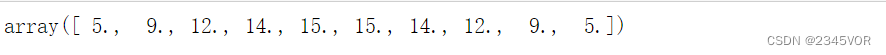B = A.todense()
x = la.solve(B, b)
x

• 1
• 2
• 3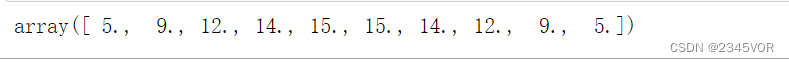N = 1000
A = sp.diags([1, -2, 1], [1, 0, -1], shape=[N, N], format='csc')
b = -np.ones(N)
B = A.todense()
%time x = sp.linalg.spsolve(A, b)

• 1
• 2
• 3
• 4
• 5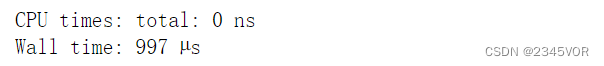%time x = la.solve(B, b)

• 1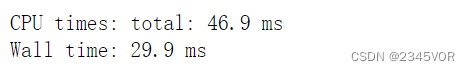# compare performance of solving Ax=b vs system size N,
# where A is the sparse matrix for the 1d poisson problem

import time

def setup(N):
A = sp.diags([1,-2,1], [1,0,-1], shape=[N, N], format='csr')
b = -np.ones(N)
return A, A.todense(), b

reps = 10
N_vec = np.arange(2, 300, 1)
t_sparse = np.empty(len(N_vec))
t_dense = np.empty(len(N_vec))
for idx, N in enumerate(N_vec):
A, A_dense, b = setup(N)
t = time.time()
for r in range(reps):
x = np.linalg.solve(A_dense, b)
t_dense[idx] = (time.time() - t)/reps
t = time.time()
for r in range(reps):
x = sp.linalg.spsolve(A, b, use_umfpack=True)
t_sparse[idx] = (time.time() - t)/reps

fig, ax = plt.subplots(figsize=(8, 4))
ax.plot(N_vec, t_dense * 1e3, '.-', label="dense")
ax.plot(N_vec, t_sparse * 1e3, '.-', label="sparse")
ax.set_xlabel(r"$N$", fontsize=16)
ax.set_ylabel("elapsed time (ms)", fontsize=16)
ax.legend(loc=0)
fig.tight_layout()

• 1
• 2
• 3
• 4
• 5
• 6
• 7
• 8
• 9
• 10
• 11
• 12
• 13
• 14
• 15
• 16
• 17
• 18
• 19
• 20
• 21
• 22
• 23
• 24
• 25
• 26
• 27
• 28
• 29
• 30
• 31
• 32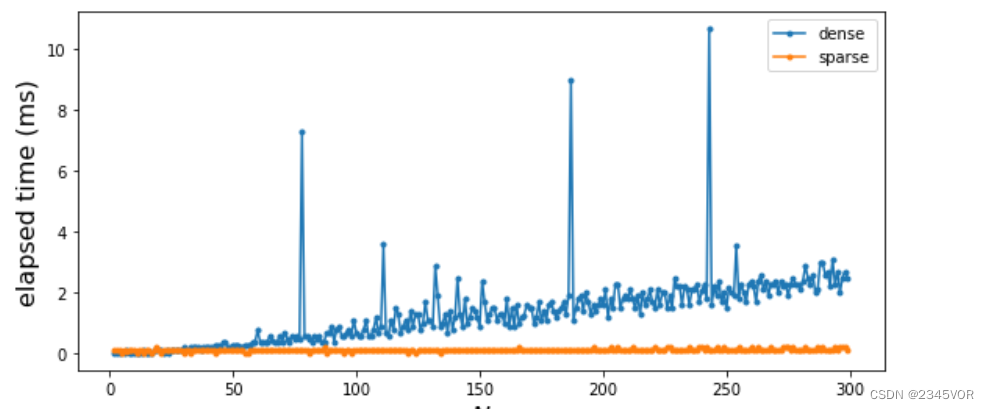### 3.4 LU分解

b

b

b求解

A

x

=

b

Ax=b

Ax=b，这将特别有用。

N = 1000
A = sp.diags([1, -2, 1], [1, 0, -1], shape=[N, N], format='csc')
b = -np.ones(N)

%time lu = sp.linalg.splu(A)

• 1
• 2
• 3
• 4
• 5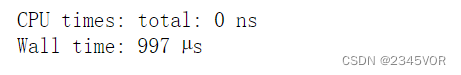lu.L, lu.U

• 1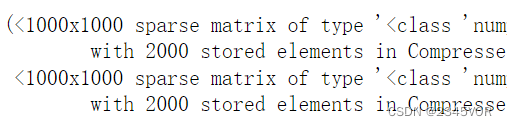A

x

=

b

Ax=b

Ax=b了。

x = lu.solve(b)

• 1

### 3.5 特征值问题

N = 10
A = sp.diags([1, -2, 1], [1, 0, -1], shape=[N, N], format='csc')

evals, evecs = sp.linalg.eigs(A, k=4, which='LM')
evals

• 1
• 2
• 3
• 4
• 5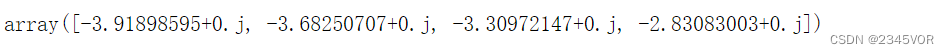sp.linalg.eigs函数返回的是一个元组，其中第一个元素是特征值数组，第二个元素是特征向量数组。

np.allclose(A.dot(evecs[:,0]), evals * evecs[:,0])  # 排除浮点数误差影响

• 1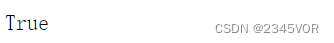N = 1000
A = sp.diags([1, -2, 1], [1, 0, -1], shape=[N, N], format='csc')
B = A.todense()
%time evals, evecs = sp.linalg.eigs(A, k=4, which='LM')

• 1
• 2
• 3
• 4%time evals, evecs = la.eig(B)

• 1